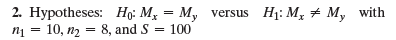×
Get Full Access to Statistics: Informed Decisions Using Data - 5 Edition - Chapter 15.5 - Problem 2
Get Full Access to Statistics: Informed Decisions Using Data - 5 Edition - Chapter 15.5 - Problem 2

×

# ?In Problems 1–8, use the Mann–Whitney test to test the given hypotheses at the = 0.05 level of significance. The independent samples were obtained raISBN: 9780134133539 240

## Solution for problem 2 Chapter 15.5

Statistics: Informed Decisions Using Data | 5th Edition

• Textbook Solutions
• 2901 Step-by-step solutions solved by professors and subject experts
• Get 24/7 help from StudySoup virtual teaching assistantsStatistics: Informed Decisions Using Data | 5th Edition

4 5 1 339 Reviews
21
2
Problem 2

In Problems 1–8, use the Mann–Whitney test to test the given hypotheses at the= 0.05 level of significance. The independent samples were obtained randomly.

2. Hypotheses: H0: Mx = My versus H1: Mx ≠ My with n1 = 10, n2 = 8, and S = 100Step-by-Step Solution:

Step 1 of 5) Use the Mann–Whitney test to test the given hypotheses at the = 0.05 level of significance. The independent samples were obtained randomly. 2. Hypotheses: H0: Mx = My versus H1: Mx ≠ My with n1 = 10, n2 = 8, and S = 100. Construct and Interpret Confidence Intervals for the Population Variance and Standard Deviation The sample variance, s2, is the best point estimate of the population variance, s2. We use the sample standard deviation, s, as the point estimate of the population standard deviation, s.* We now develop a method for constructing a confidence interval for the population variance. Suppose we take a simple random sample of size n from a population that is normally distributed with mean m and standard deviation s; then x2 = 1n - 12 s2 s2 follows a chi-square distribution with n - 1 degree of freedom. Therefore, 11 - a2 # 100% of the values of x2 will lie between x1 - a > 2 2 and xa>2 2 .

Step 2 of 2

## Discover and learn what students are asking

Calculus: Early Transcendental Functions : Cylindrical and Spherical Coordinates
?In Exercises 1-6, convert the point from cylindrical coordinates to rectangular coordinates. (-7, 0, 5)

Calculus: Early Transcendental Functions : Second-Order Nonhomogeneous Linear Equations
?Verifying a Solution In Exercises 1-4,verify the solution of the differential equation. Solution D

Statistics: Informed Decisions Using Data : Comparing Three or More Means (One-Way Analysis of Variance)
?Births by Day of Week An obstetrician knew that there were more live births during the week than on weekends. She wanted to determine whether the mean

Unlock Textbook Solution